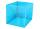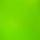# Square + right triangle - examples

1. Prism 4 sidesThe prism has a square base with a side length of 3 cm. The diagonal of the sidewall of the prism/BG/is 5 cm. Calculate the surface of this prism in cm square and the volume in liters
2. GardenArea of square garden is 6/4 of triangle garden with sides 56 m, 35 m and 35 m. How many meters of fencing need to fence a square garden?
3. SquarePoints A[-5,-6] and B[7,-1] are adjacent vertices of the square ABCD. Calculate the area of the square ABCD.
4. LogsTrunk diameter is 52 cm. Is it possible to inscribe a square prism with side 36 cm?
5. Square and circlesSquare with sides 61 mm is circumscribed and inscribed with circles. Determine the radiuses of both circles.
6. Square diagonalCalculate length of the square diagonal if the perimeter is 476 cm.
7. PrismRight angle prism, whose base is right triangle with leg a = 7 cm and hypotenuse c = 10 cm has same volume as a cube with an edge length of 1 dm. a) Determine the height of the prism b) Calculate the surface of the prism c) What percentage of the cube
8. StepsHow many steps you save if you go square estate for diagonal (crosswise), rather than circumvent on the two sides of its perimeter with 307 steps.
9. InfinityIn a square with side 18 is inscribed circle, in circle is inscribed next square, again circle and so on to infinity. Calculate the sum of area of all these squares.
10. OctagonWe have a square with side 84 cm. We cut the corners to make his octagon. What will be the side of the octagon?
11. Recursion squaresIn the square ABCD is inscribed a square so that its vertices lie at the centers of the sides of the square ABCD.The procedure of inscribing square is repeated this way. Side length of square ABCD is a = 22 cm. Calculate: a) the sum of perimeters of all
12. Square diagonalCalculate the length of diagonal of the square with side a = 23 cm.
13. 4side pyramidCalculate the volume and surface of 4 side regular pyramid whose base edge is 4 cm long. The angle from the plane of the side wall and base plane is 60 degrees.
14. Square 2Points D[10,-8] and B[4,5] are opposed vertices of the square ABCD. Calculate area of the square ABCD.
15. Tetrahedral pyramidCalculate the volume and surface of the regular tetrahedral pyramid if content area of the base is 20 cm2 and deviation angle of the side edges from the plane of the base is 60 degrees.
16. SquareCalculate area of the square with diagonal 64 cm.
17. TaskI have homework. The cube has an edge 7 cm long and I must find wall and body diagonal.
18. Square pyramidCalculate the volume of the pyramid with the side 5cm long and with a square base, side-base has angle of 60 degrees.
19. Cube wallCalculate the cube's diagonal diagonal if you know that the surface of one wall is equal to 36 centimeters square. Please also calculate its volume.
20. Square2Side of the square is a = 6.2 cm, how long is its diagonal?

Do you have an interesting mathematical example that you can't solve it? Enter it, and we can try to solve it.

To this e-mail address, we will reply solution; solved examples are also published here. Please enter e-mail correctly and check whether you don't have a full mailbox.

See also our right triangle calculator.